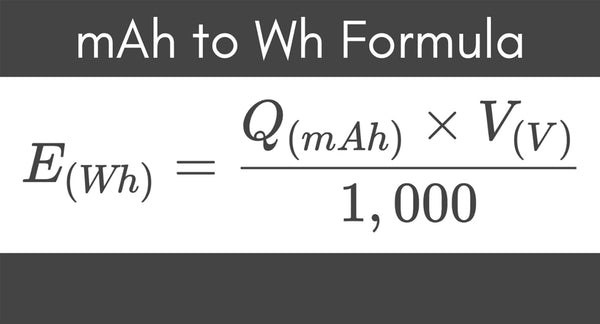mAh to Watts Calculator: Why Do I Need It? - ShopSolar.com

# mAh to Watts Calculator

If you’re looking for an mAh to watts calculator, you’ve come to just the right place. We’re on a mission to make solar simple.

We’re going to explain how to do this calculation as well as why it’s useful in terms of your solar power system.

We’ll also give you an example to illustrate how you should use the formula. We know that seeing math can be frightening for some, but we’re going to make it as simple as possible!

## What Is mAh?mAH refers to milliamp hours. It’s one-thousandth of an ampere-hour. Amps measure the current of a circuit.

Milliamp hours (mAh) and ampere-hours (Ah) measure the energy a battery holds and how long it can run before the battery dies.

Basically, milliamp hours measure electric power over time.

In terms of your solar power system, this measure tells you how long your inverter will last before dying.

## Why Is it Useful to Convert mAh to Watts?

To understand this, let’s go over what watts are. One watt is one unit of electrical power. It’s what the power your inverter stores is measured in.

Using a mAh to Watts calculator goes hand in hand with understanding how many watts your household uses so you can invest in a solar system large enough to meet your needs.

It also measures the power your appliances use, so by knowing how many watts you have available to use, you can know how many appliances you can connect to your inverter.

This knowledge also helps ensure you don’t overload your inverter and degrade the battery over time. You should never run your inverter below 50% capacity, and this knowledge helps you make sure you never do.

Depending on where you live, the power output of your system is going to be different. You can use the NREL’s PV watt calculator to find an accurate estimate of what you can expect to produce.

## Convert mAh to Watts

The formula we’re going to use is: mAh x V / 1,000 = Wh

It looks a bit intimidating, we know. It’s quite simple though. Here’s what the symbols stand for:

Milliamp-hours multiplied by volts divided by 1,000 equals watt-hours.

There is one watt in every watt-hour. This means the units are equal to each other, and we can read watt hours as watts.Now that we understand the variables in the formula, let’s go over an example by plugging in values.

We’re going to work with the assumption that you solar inverter has a voltage rating of 48 V. However, if yours is different, just plug in that value.

We’ll also assume you’re working with 180 Ah, which is relatively standard. This is 180,000 mAh.

If you’re not sure of the voltage or ampere-hour of your inverter, take a look at the side of it. There’s usually a sticker with this information in that area.

mAh x V / 1,000 = Wh

180,000 x 48 / 1,000 = Wh

Wh = 8,640.

This means your wattage is 8,640 W.

Knowing this information, it’s easy to extrapolate these values out to other units. For example, you can take the number worked out in this equation and easily find out an mA to watts conversion.

## Conclusion

If you’re looking to do some more electrical calculations to understand your solar power system better, have a look at our 115 volts to watts calculator and Va to Watts chartIf you’re unsure of your needs and are looking to invest in a solar setup, we’re here to help. We put together complete solar panel kits for home, so you don’t have to do all the digging around to figure out which parts go best together.

If you don’t feel that any of these options are a good fit, get in touch with us. We’ll assess your needs and direct you toward the right products.

Did You Find Our Blog Helpful? Then Consider Checking: